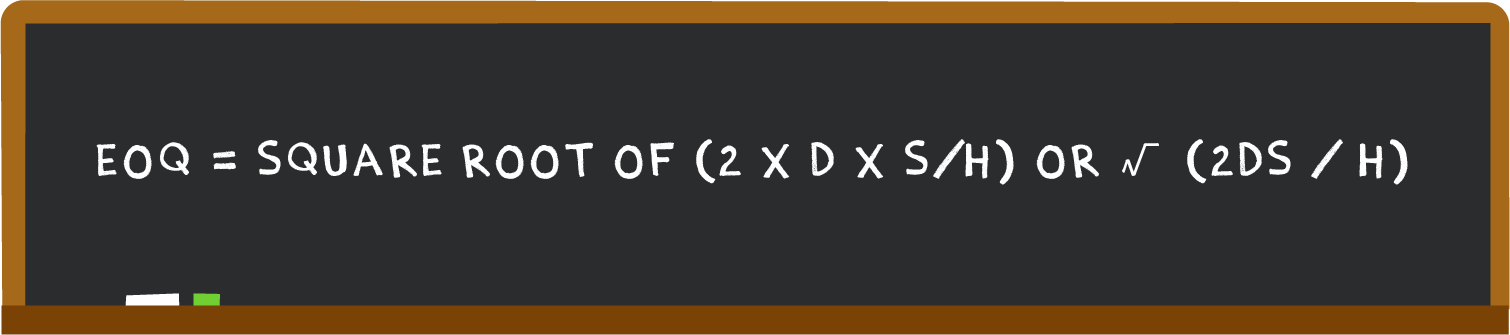inventory

# What is economic order quantity and how do you calculate it?

The economic order quantity (EOQ) is the order quantity that helps minimize holding costs and order costs for your business. Use the EOQ formula to start optimizing your inventory costs.

## What is Economic Order Quantity?

Economic order quantity (EOQ), refers to the optimum amount of an item that should be ordered at any given point in time, such that the total annual cost of carrying and ordering that item is minimized. EOQ is also sometimes known as the optimum lot size. Simply put – how much product should you purchase to maintain a cost-efficient supply chain?

## Why is the EOQ important?

The EOQ helps companies minimize the cost of ordering and holding inventory. As explained by the economic concept known as economies of scale, the cost per unit of ordering a product falls, the larger the total quantity of the order. However, the larger the total quantity of an order, the higher the cost to hold and carry your inventory.

## EOQ formula

• Determine the demand in units
• Determine the order cost (incremental cost to process and order)
• Determine the holding cost (incremental cost to hold one unit in inventory)
• Multiply the demand by 2, then multiply the result by the order cost.
• Divide the result by the holding cost.
• Calculate the square root of the result to obtain EOQ.

In short: EOQ = square root of (2 x D x S/H) or √ (2DS / H)Where:

• D represents demand, or how many units of product you need to buy.
• S represents setup cost.
• H represents the holding fee or storage cost per unit of product.

Example of calculating EOQ

Meet Matt. Matt runs a men’s clothing line. Matt needs to buy 12,000 shirts per year to fulfill demand (D). He incurs a setup cost of \$100 (S) and a holding fee (H) of \$16 per shirt. He needs to know his EOQ.

Plugging those numbers into the EOQ formula, you get:

EOQ = √ (2 x 12,000 x 100/16)

EOQ = √ \$3,456,000 / \$16

EOQ = √216,000

EOQ = 465 units (rounded up to the nearest whole unit)

The EOQ is usually used to set the reorder point within your inventory management workflows. Together, these metrics tell you when to place an order (reorder point) and how much order to place (EOQ formula). This prevents you from carrying too much deadstock or facing stockouts.

Minimize inventory cost.

Although the formula to calculate EOQ is simple when applied to a single product, calculating it manually for a whole inventory can be time-consuming and there is a margin for human error. Using inventory tools such as QuickBooks Commerce’s free EOQ calculator can help you save valuable time and what’s more- it's free!

### Are you looking for an effortless inventory management solution?

Save time and money by signing up for a free trial of QuickBooks Commerce today!

### Take control with Advanced Inventory

Whether you sell five or 50,000 products, QuickBooks Enterprise puts the tools you need for efficient, profitable inventory management right at your fingertips.### Get the latest to your inbox

Relevant resources to help start, run, and grow your business, delivered twice a month.

Thanks for subscribing.

Relevant resources to help start, run, and grow your business, delivered twice a month.